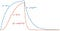The Potassium Conductance

Yesterday’s post led me to reflect on how Russ Hobbie and I describe the potassium conductance of a nerve membrane in Chapter 6 of Intermediate Physics for Medicine and Biology. In the Hodgkin and Huxley model, the time dependence of the potassium conductance is proportional to n^4(t), where n is a dimensionless variable called the potassium gate that takes values from zero (potassium channels are closed) to one (channels open).

6.13.2 Potassium Conductance

Hodgkin and Huxley wanted a way to describe their extensive voltage-clamp data, similar to that in Figs. 6.34 and 6.35,. with a small number of parameters. If we ignore the small nonzero value of the conductance before the clamp is applied, the potassium conductance curve of Fig. 6.34 is reminiscent of exponential behavior, such as g_K(v,t) = g_K(v) (1 — e^(-t/tau(v))), with both g_K(v) and τ(v) depending on the value of the voltage. A simple exponential is not a good fit. Figure 6.36 shows why. The curve (1 — e^(-t/tau)) starts with a linear portion and is then concave downward. The potassium conductance in Figs. 6.34 and 6.35 is initially concave upward. The curve (1 — e^(-t/tau))^4 in Fig. 6.36 more nearly has the shape of the conductance data.

This is all correct, but the story has another part. Hodgkin and Huxley focus on this missing part in their 1952 paper “The Components of Membrane Conductance in the Giant Axon of Loligo,” Journal of Physiology, Volume 116, Pages 473–496.

The experiment shows that whereas the potassium conductance rises with a marked delay it falls along an exponential type of curve which has no inflexion corresponding to that on the rising phase. [my italics]

Russ and I showed in Fig. 6.36 how our model predicts that the potassium conductance rises with a marked delay, but we didn’t check if it falls with “inflexion.” Will the fall have a sigmoidal shape like the rise does, or will it be abrupt like Hodgkin and Huxley observed?

To check, I derived a simple exponential solution for n( t) during both the rising phase (0 < t < 4 τ) and the falling phase (t > 4 τ). This is a toy model for an experiment in which we clamp the voltage at a depolarizing value for a duration of 4 τ, and then return it to rest.

This may look like a complicated expression, but it’s simply the solution to the differential equation

when n(0) = 0,

and τ is independent of time.

I made a plot of n(t) and n4(t), which is an extension of IPMB’s Fig. 6.36 to longer times.A plot of n(t) (blue) and n^4(t) (red) versus time. This is an extension of Fig. 6.36 in IPMB.

The gate itself, n(t) (blue), rises exponentially and then falls exponentially, with no hint of sigmoidal behavior. However, n^4(t) (red) rises with a sigmoidal shape but then falls exponentially. This is exactly what Hodgkin and Huxley observed experimentally.

The simple toy model that Russ and I use to illustrate the potassium conductance works better than we realized!

Originally published at http://hobbieroth.blogspot.com.

Professor of Physics at Oakland University and coauthor of the textbook Intermediate Physics for Medicine and Biology.